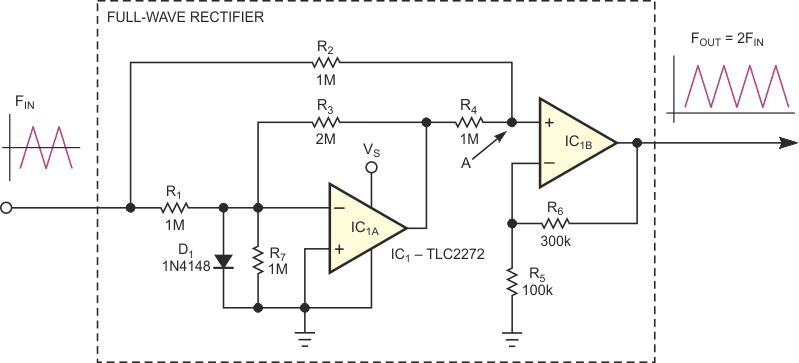# Frequency doubler operates on triangle wave

## Texas Instruments TLC2272

Frequency multipliers typically work with square waves. However, the circuit in Figure 1 performs frequency multiplication on triangle waveforms and maintains the input's amplitude and uniformity.Figure 1. This circuit performs frequency multiplication on triangle waveforms.

The general idea is to apply the triangle waveform to any full-wave rectifier. The output is then a triangle wave with twice the input frequency plus some dc bias. You then can remove the dc bias using a simple highpass filter or by shifting the bias level with another op amp. You can continually repeat this trick to obtain a frequency series of 2×FIN, 4×FIN, 8×FIN, and so on.

The actual circuit in Figure 1 uses a single-supply, dual op amp to help perform the rectification. When the input is the negative half-wave signal, IC1A works as a regular inverting amplifier which R1 and R3 set to a gain of 2. The circuit then produces the algebraic sum, with a coefficient of 0.5, of the positive output signal of IC1A and the negative input signal at summing point A. After IC1B amplifies the signal by 4, according to the values of R5 and R6, the output signal has the same peak-to-peak amplitude as the input, but with a positive sign.

When the input goes positive, the only path to summing point A is through R2. In this case, IC1A saturates, and its output sits close to ground potential. Good rail-to-rail op amps can swing within a few millivolts of ground. Thus, the signal at A remains positive, but divided by 2 due to the voltage divider of R2 and R4. IC1B restores the original amplitude of the signal. Thus, the output is a triangle wave equal in peak-to-peak amplitude to but twice the frequency of the input.

D1 and R7 are optional; they improve the dynamic performance of the circuit by preventing the input stage overload and the impact of IC1A’s input capacitance when the input signal goes positive. If the input frequency doesn't exceed 1 kHz, you can omit D1 and R7. For higher frequencies, keep these components. Moreover, you can proportionally reduce the values of all resistors. Also, you can reduce the value of R7 to 10 or 20% of R1, but this change may cause dc bias of the output and adversely affect the circuit's accuracy.

The component values and op amp in Figure 1 make this circuit applicable to low-frequency-range applications, such as a frequency (octave) synthesizer for electro-musical instruments. For applications having frequencies higher than 20 kHz, you must choose a faster op amp and, possibly, a different rectifier topology.

In Figure 1’s full-wave rectifier topology, the input impedance differs for positive and negative waveforms. To reduce this difference, you can connect a constant resistor between the input pin and ground. When coupling numerous multiplying stages, the simple RC, highpass filter also helps minimize the impedance asymmetry. For this filter, choose as small a resistor as necessary to reduce the impedance asymmetry between positive- and negative-going signals to a reasonable level and then choose the capacitor for the filter frequency you choose.

## Materials on the topic

EDNYou may have to register before you can post comments and get full access to forum.
 User Name Remember Me? Password